Next: Resonance and the Transfer Up: Sinusoidal Sources and Complex Previous: Inductive Impedance

## Combined Impedances

We now know the impedance for each of our passive circuit elements: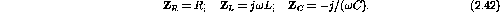The equivalent impedance of a circuit can be obtained by using the following rules for combining impedances.

In series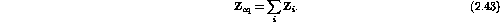In parallel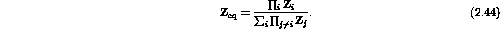Appealing to the complex notation we can write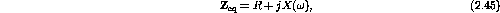where R is the resistance and X is called the reactance (always a function of).

For a series combination of R, L and C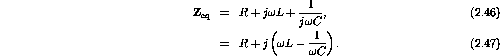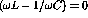gives a special frequency,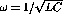.

Example: An inductor and capacitor in parallel form the tank circuit shown in figure 2.5.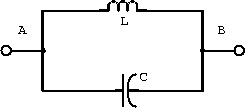Figure 2.5:  Tank circuit with inductor and capacitor.

1. Determine an expression for the impedance of this circuit.

The impedance of an inductor and a capacitor are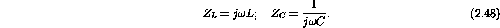Combining the impedances in parallel gives2. What is the impedance when?

Substituting this value forinto the above result gives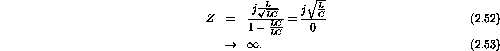Example: The tank circuit schematic shown in figure 2.6 results from the use of a real inductor.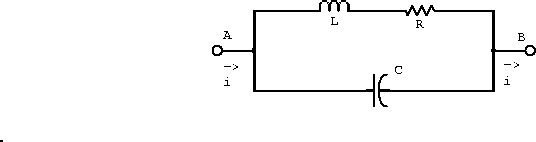Figure 2.6:  Tank circuit with real inductor

1. Find an expression for the impedance of this circuit.

The impedance of an inductor, capacitor and resistor are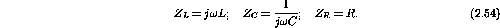The resistor and inductor are in series and this combination of impedance is in parallel with the capacitor. Combining the impedances gives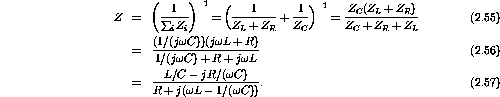2. If L=1H,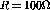, and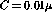F, what is the impedance when?

Substituting this value forinto the above equation gives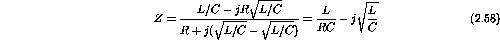Substituting the numerical values for the inductance, resistance and capacitance gives3. What is the impedance whenis very small?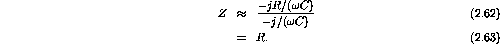4. What is the phase angle between the voltage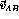andat resonance and at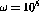rad/s?

Rationalizing the denominator of the impedance gives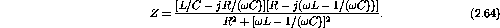Taking the real and imaginary components gives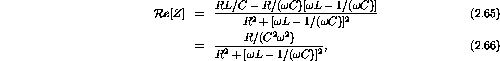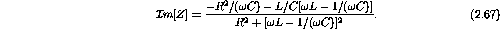The inverse tangent of the ratio of the imaginary to real parts isThere is a resonance at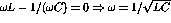and hence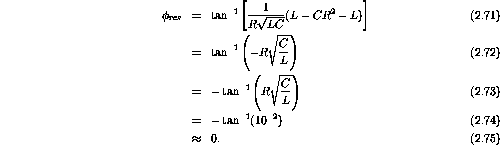Atrad/s.Next: Resonance and the Transfer Up: Sinusoidal Sources and Complex Previous: Inductive Impedance

Doug Gingrich
Tue Jul 13 16:55:15 EDT 1999